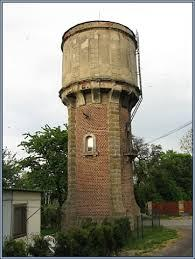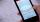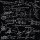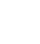# Water reservoir

The cuboid reservoir contains 1900 hectoliters of water and the water height is 2.5 m. Determine the dimensions of the bottom where one dimension is 3.2 m longer than the second one.

Correct result:

a =  7.26 m
b =  10.46 m

#### Solution:

$V = abc = 1900 \ hl = 190 \ m^3 \ \\ 190 = 2.5 ab \ \\ ab = 76 \ \\ b = 3.2+a \ \\ \ \\ a(3.2+a) = 76 \ \\ a^2 + 3.2 a - 76 = 0 \ \\ \ \\ x_{1,2} = \dfrac{ -b \pm \sqrt{ D } }{ 2a } = \dfrac{ -3.2 \pm \sqrt{ 314.24 } }{ 2 } \ \\ x_{1,2} = -1.6 \pm 8.86340792246 \ \\ x_{1} = 7.26340792246 \ \\ x_{2} = -10.4634079225 \ \\ \ \\ a = 7.26 \ \text{m}$We would be pleased if you find an error in the word problem, spelling mistakes, or inaccuracies and send it to us. Thank you!Tips to related online calculators
Looking for help with calculating roots of a quadratic equation?
Do you have a linear equation or system of equations and looking for its solution? Or do you have quadratic equation?

## Next similar math problems:

• DiscriminantDetermine the discriminant of the equation: ?
• Reciprocal equation 2Solve this equation: x + 5/x - 6 = 4/11
• Solve 3Solve quadratic equation: (6n+1) (4n-1) = 3n2Find the roots of the quadratic equation: 3x2-4x + (-4) = 0.
• FractionFor what x expression ? equals zero?
• Cinema 4In cinema are 1656 seats and in the last row are 105 seats , in each next row 3 seats less. How many are the total rows in cinema?
• EquationHow many real roots has equation ? ?
• Function 3Function f(x)=a(x-r)(x-s) the graph of the function has x- intercept at (-4, 0) and (2, 0) and passes through the point (-2,-8). Find constant a, r, s.
• Expression with powersIf x-1/x=5, find the value of x4+1/x4Quadratic equation ? has roots x1 = -26 and x2 = -86. Calculate the coefficients b and c.
• RootsDetermine the quadratic equation absolute coefficient q, that the equation has a real double root and the root x calculate: ?
• BirthdaysIn the classroom, students always give candy to their classmates on their birthdays. The birthday person always gives each one candy, and he does not give himself. A total of 650 candies were distributed in the class per year. How many students are in the
• EquationEquation ? has one root x1 = 8. Determine the coefficient b and the second root x2.
• Roots countSubstitute the numbers/0,1,2,3/into the equation as x: (x - 1) (x - 3) (x + 1) = 0 Which of them is its solution? Is there another number that solves this equation?
• AlgebraX+y=5, find xy (find the product of x and y if x+y = 5)
• The productThe product of a number plus that number and its inverse is two and one-half. What is the inverse of this number
• Stones 3Simiyu and Nasike each collected a number of stones in an arithmetic lesson. If Simiyu gave Nasike 5 stones, Nasike would have twice as many stones as Simiyu. If initially, Simiyu had five stones less than Nasike how many stones did each have?